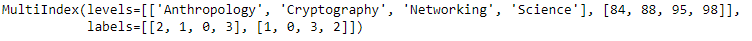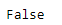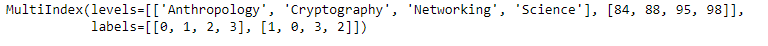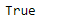# Python | Pandas MultiIndex.is_lexsorted()

• Last Updated : 24 Dec, 2018

Python is a great language for doing data analysis, primarily because of the fantastic ecosystem of data-centric python packages. Pandas is one of those packages and makes importing and analyzing data much easier.

Pandas` MultiIndex.is_lexsorted()` function return `True` if the labels are lexicographically sorted. Otherwise the function return `False` indicating the labels are not lexicographically sorted.

Syntax: MultiIndex.is_lexsorted()

Parameters : None

Returns : boolean

Example #1: Use `MultiIndex.is_lexsorted()` function to check if the MultiIndex labels are lexicographically sorted or not.

 `# importing pandas as pd``import` `pandas as pd`` ` `# Create the MultiIndex``midx ``=` `pd.MultiIndex.from_arrays([[``'Networking'``, ``'Cryptography'``,``                                     ``'Anthropology'``, ``'Science'``], ``                                             ``[``88``, ``84``, ``98``, ``95``]])`` ` `# Print the MultiIndex``print``(midx)`

Output :Now let’s check if the labels in the MultiIndex are lexicographically sorted or not.

 `# check if labels are sorted or not``midx.is_lexsorted()`

Output :As we can see in the output, the function has returned false indicating that the labels in the MultiIndex are not lexicographically sorted.

Example #2: Use `MultiIndex.is_lexsorted()` function to check if the MultiIndex labels are lexicographically sorted or not.

 `# importing pandas as pd``import` `pandas as pd`` ` `# Create the MultiIndex``midx ``=` `pd.MultiIndex.from_arrays([[``'Anthropology'``, ``'Cryptography'``, ``                                         ``'Networking'``, ``'Science'``], ``                                               ``[``88``, ``84``, ``98``, ``95``]])`` ` `# Print the MultiIndex``print``(midx)`

Output :Now let’s check if the labels in the MultiIndex are lexicographically sorted or not.

 `# check if labels are sorted or not``midx.is_lexsorted()`

Output :As we can see in the output, the function has returned true indicating that the labels in the MultiIndex are lexicographically sorted.

My Personal Notes arrow_drop_up